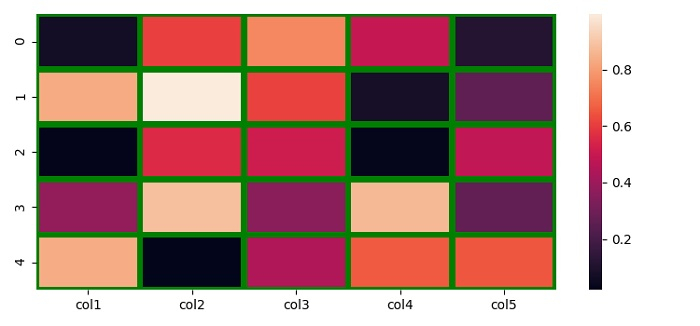# How to make custom grid lines in Seaborn heatmap?

To make custom grid lines in Seaborn heatmap, we can use linewidths and linecolor values in the heatmap() method.

## Steps

• Set the figure size and adjust the padding between and around the subplots.

• Create a Pandas dataframe with 5 columns.

• Use heatmap() method to plot the rectangular data as a color-encoded matrix.

• To display the figure, use show() method.

## Example

import seaborn as sns
import pandas as pd
import numpy as np
import matplotlib.pyplot as plt

plt.rcParams["figure.figsize"] = [7.50, 3.50]
plt.rcParams["figure.autolayout"] = True

df = pd.DataFrame(np.random.random((5, 5)), columns=["col1", "col2", "col3", "col4", "col5"])

sns.heatmap(df, linewidths=4, linecolor='green')

plt.show()

## Output